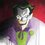# Every Prime Except Two Can be Written as a Difference of Two Squares

$\mathbf{Theorem:}$ every prime except $2$ can be written as a difference of two square numbers

We have to find two integers $a$ and $b$ such that $a^{2} - b^{2} = p ..... \left( i \right )$ for a prime $p$

now we can rewrite equation $\left ( i \right )$ as,

$a^{2} - b^{2} = p$ $\Rightarrow (a - b)(a + b) = p$

now as $p$ is a prime number we can't write $p$ as a product of two integers except for $1$ and $p$ itself. from this, we get that, $a - b = 1 ....... \left( ii \right )$ $a + b = p ....... \left( iii \right)$

solving equation $(ii)$ and $(iii)$ , we get $a = \frac{p + 1}{2}$ and $b = \frac{p - 1}{2}$.

notice that $a$ and $b$ are integers if $p$ is an odd number. Since all primes except for $2$ are odd, our proof is complete for every odd primes.

now we have to proof for the even primes, which is only $2$. again notice that $a$ and $b$ can't be integer if $p$ is an even number. so we can't write $p$ as $a^{2} - b^{2}$ if $p$ is an even integer.

so from here, we can only deduce that we can write $p$ as $a^{2} - b^{2}$ for any prime except for $2$

$.$ $.$

a friend of mine claims my proof is incomplete but I can't find it. can you point it where?Note by Tasmeem Reza
3 years, 6 months ago

This discussion board is a place to discuss our Daily Challenges and the math and science related to those challenges. Explanations are more than just a solution — they should explain the steps and thinking strategies that you used to obtain the solution. Comments should further the discussion of math and science.

When posting on Brilliant:

• Use the emojis to react to an explanation, whether you're congratulating a job well done , or just really confused .
• Ask specific questions about the challenge or the steps in somebody's explanation. Well-posed questions can add a lot to the discussion, but posting "I don't understand!" doesn't help anyone.
• Try to contribute something new to the discussion, whether it is an extension, generalization or other idea related to the challenge.

MarkdownAppears as
*italics* or _italics_ italics
**bold** or __bold__ bold
- bulleted- list
• bulleted
• list
1. numbered2. list
1. numbered
2. list
Note: you must add a full line of space before and after lists for them to show up correctly
paragraph 1paragraph 2

paragraph 1

paragraph 2

[example link](https://brilliant.org)example link
> This is a quote
This is a quote
    # I indented these lines
# 4 spaces, and now they show
# up as a code block.

print "hello world"
# I indented these lines
# 4 spaces, and now they show
# up as a code block.

print "hello world"
MathAppears as
Remember to wrap math in $$ ... $$ or $ ... $ to ensure proper formatting.
2 \times 3 $2 \times 3$
2^{34} $2^{34}$
a_{i-1} $a_{i-1}$
\frac{2}{3} $\frac{2}{3}$
\sqrt{2} $\sqrt{2}$
\sum_{i=1}^3 $\sum_{i=1}^3$
\sin \theta $\sin \theta$
\boxed{123} $\boxed{123}$

Sort by:

If I'm not mistaken, your proof is backwards; that is, you started by assuming that every odd prime can be written as a difference of perfect squares, and then verifying that $a$ and $b$ are solutions. Essentially, what you have proven is that "if $p = a^2 - b^2$, then $a = (p + 1)/2$ and $b = (p - 1)/2$ is a solution." This is not quite the same as, though very closely related to, proving "there exist $a$ and $b$ such that $p = a^2 - b^2$." The quick fix to this problem would be to reverse your steps.

However, I believe you have successfully shown that 2 cannot be written in the form $a^2 - b^2$ by contradiction. So, I would guess that your friend was referring to the above issue.

- 3 years, 6 months ago

Indeed. Even after we found a sequence of steps that lead to a solution/proof, we have to be careful with how it is written up, and ensure that the statements flow in the direction that we desire. It is very common to end up with implication in the opposite direction of what is desired.

Staff - 3 years, 5 months ago